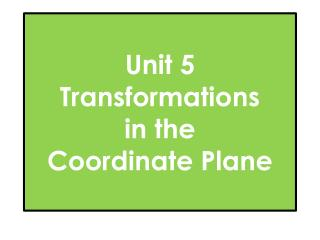Download PresentationUnit 5 Transformations in the Coordinate Plane

Unit 5 Transformations in the Coordinate Plane - PowerPoint PPT Presentation

Unit 5 Transformations in the Coordinate Plane. Translations. Reflections. Rotations. Question 1. The top shape (pre image) has been translated to the bottom shape (image). Which rule represents the translation? a) c ) b) d). Question 2. If the result ofI am the owner, or an agent authorized to act on behalf of the owner, of the copyrighted work described.
Download PresentationUnit 5 Transformations in the Coordinate Plane

Download Policy: Content on the Website is provided to you AS IS for your information and personal use and may not be sold / licensed / shared on other websites without getting consent from its author.While downloading, if for some reason you are not able to download a presentation, the publisher may have deleted the file from their server.

- - - - - - - - - - - - - - - - - - - - - - - - - - E N D - - - - - - - - - - - - - - - - - - - - - - - - - -
Presentation Transcript
1. Unit 5Transformations in theCoordinate Plane

2. Translations

3. Reflections

4. Rotations

5. Question 1 The top shape (pre image) has been translated to the bottom shape (image). Which rule represents the translation? a) c) b) d)

6. Question 2 If the result of (x, y)→(x – 4, y + 3) is A’(-2, 8), what is the pre-image, or A?

7. Question 3 If A(4, -9) is translated using the rule, (x, y)→(x+5, y-7) what is the image, or A’?

8. Question 4 Which best describes the transformation that occurs in the graph? a) c) b) d)

9. Question 5 A triangle in the coordinate place has coordinates of (2, 3), (-4, -5), and (-2, 4). It is reflected about the x-axis. What are the new coordinates?

10. Question 6 The figure on the left has been transformed to the figure on the right. Which transformation does this show? a) c) b) d)

11. Question 7 Reflect the figure across x=1. What is the image of T?

12. Question 8 The figure is transformed as shown in the diagram. Describe the transformation a) c) b) d)

13. Question 9 Rotate the point K(5,-6) 270 Clockwise about the origin. what is the image, or K’?

14. Question 10 If C(9,4) is reflected over the y-axis, then reflected over the line y=-x. What is the final image, or C”?

15. Question 11 If B(2,6) is translated using the rule (x, y)→(x +7, y -4), and then rotated 180about the origin. What is the final image, or B”?

16. Question 12 A reflection of triangle abc to triangle a’b’c’ is shown. Which rule represents this reflection? a) c) b) d)

17. Question 13 Which transformation will be equivalent to rotating a figure 180 counterclockwise? a) c) b) d)

18. Question 14 Describe the transformation a) c) b) d)

19. Answers to MC Practice Back 6. A 7. D 8. B 9. B Front • B • C • D • C • C